﻿ 基于非精确单调与非单调线搜索的全波形反演

# 基于非精确单调与非单调线搜索的全波形反演A Full Waveform Inversion Based on Inexact Monotone and Non-Monotone Line Search

Abstract: In the mathematical physics inverse problem, full waveform inversion is a seismic imaging method with high resolution. However, its highly nonlinearity and ill-posedness of the misfit functional make it easy to fall into the local minima. For the multiple local minima problem of the full-waveform inversion, a comprehensive comparison is made based on the globalization strategy of approximate monotone and non-monotone line search, and a full-waveform inversion algorithm is established based on the non-monotone line search globalization strategy and Newton method. To efficiently solve the large-scale linear equations of Newton method, a conjugate gradient method is constructed based on the Lanczos diagonalization method to approximately solve Newton equation, and a matrixfree truncated Newton inversion algorithm is established. In order to further improve the computational efficiency of the truncated Newton inversion method, an efficient method to efficiently calculate matrix vector products is derived based on the adjoint method. The numerical simulation is carried out based on Sigsbee model. The numerical results show that, without increasing compu- tational loads, the performance of the truncated Newton inversion method based on the non-monotone line search is better than that of the method based on the monotone line search in terms of convergent speed and computational efficiency.

1. 引言

20世纪80年代，Lailly，Tarantola等   分别提出了时间域地震波形反演方法，为全波形反演研究奠定了理论基础。全波形反演(Full Waveform Inversion，简写为FWI)能够充分利用地震波场的全部信息，极大地提高了地震成像的分辨率 。FWI通过拟合模拟数据与观测数据，使得合成波场与观测数据残差达到最小。

$f\left(m\right)=\frac{1}{2}\underset{s=1}{\overset{{N}_{s}}{\sum }}{‖{u}_{s}\left(m\right)-{d}_{s}‖}_{2}^{2}.$ (1)

$A\left(m\right){u}_{s}={q}_{s}.$ (2)

2. 理论与方法

2.1. 截断牛顿法

$\mathrm{min}f\left(m\right),$

$\varphi \left(\delta m\right)=f\left({m}_{k}\right)+{\left(\nabla f\left({m}_{k}\right)\right)}^{\text{T}}\delta m+\frac{1}{2}{\left(\delta m\right)}^{\text{T}}{H}_{k}\delta m,$

$\nabla f\left({m}_{k}\right)+{H}_{k}\delta m=0.$ (3)

$\delta m=-{H}_{k}^{-1}\nabla f\left({m}_{k}\right).$

${m}_{k+1}={m}_{k}+{\alpha }_{k}\delta m,$ (4)

FWI是大规模反演问题，采用矩阵分解方法直接求解牛顿方程(3)是不现实的。一方面，Hessian矩阵 ${H}_{k}$ 通常无法显式计算；另一方面，即便获得了 ${H}_{k}$，采用直接法求解(3)也是不切实际的。因此，通常用CG法来近似求解牛顿方程(3)，该方法的最大优点是在求解过程中只需要计算Hessian矩阵与向量的乘积。该类方法称为截断牛顿法(或不精确牛顿法)。算法1所示为一般截断牛顿型方法的算法描述。

${\left(\nabla f\left({m}_{k}\right)\right)}^{\text{T}}\delta m<0,$

${\left(\nabla f\left({m}_{k}\right)\right)}^{\text{T}}\delta m\le -c{‖\nabla f\left({m}_{k}\right)‖}^{2},$

2.2. 单调线搜索方法

$f\left({m}_{k}\right)-f\left({m}_{k}+{\alpha }_{k}\delta m\right)>0.$

$f\left({m}_{k+1}\right)\le f\left({m}_{k}\right)+{\sigma }_{1}{\alpha }_{k}{\left(\nabla f\left({m}_{k}\right)\right)}^{\text{T}}\delta m,$ (5)

${\left(\nabla f\left({m}_{k+1}\right)\right)}^{\text{T}}\delta m\ge {\sigma }_{2}{\left(\nabla f\left({m}_{k}\right)\right)}^{\text{T}}\delta m.$ (6)

$f\left({m}_{k+1}\right)\le f\left({m}_{k}\right),k\ge 1.$

2.3. 非单调线搜索方法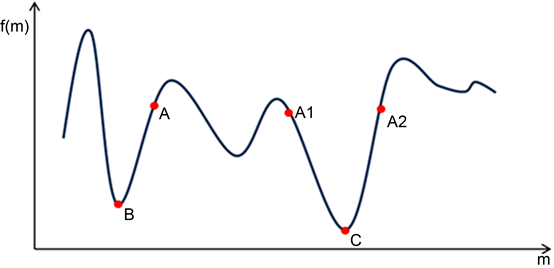Figure 1. Multiple minima for one dimension

$f\left({m}_{k+1}\right)\le {C}_{k}+{\sigma }_{1}{\alpha }_{k}{\left(\nabla f\left({m}_{k}\right)\right)}^{\text{T}}\delta m.$ (7)

${C}_{0}=f\left({m}_{0}\right),$

${Q}_{k+1}={\eta }_{k}{Q}_{k}+1,$ (8)

${C}_{k+1}=\frac{{\eta }_{k}{Q}_{k}{C}_{k}+f\left({m}_{k+1}\right)}{{Q}_{k+1}}.$

${B}_{k}=\frac{1}{k+1}\underset{i=0}{\overset{k}{\sum }}{f}_{i},{f}_{i}=f\left({m}_{i}\right),$

2.4. Lanczos对角化法

${H}_{k}\delta m=-\nabla f\left({m}_{k}\right).$

${v}_{0}\equiv 0,{\beta }_{1}{v}_{1}=-\nabla f\left({m}_{k}\right),l=1$

${p}_{l}={H}_{k}{v}_{l},{\alpha }_{l}={v}_{l}^{\text{T}}{p}_{l},$

${\beta }_{l+1}{v}_{l+1}={p}_{l}-{\alpha }_{l}{v}_{l}-{\beta }_{l}{v}_{l-1},l>1$

$\underset{_}{{T}_{l}}\equiv \left[\begin{array}{cccc}{\alpha }_{1}& {\beta }_{2}& & \\ {\beta }_{2}& {\alpha }_{2}& \ddots & \\ & \ddots & \ddots & {\beta }_{l}\\ & & {\beta }_{1}& {\alpha }_{l}\\ & & & {\beta }_{l+1}\end{array}\right].$

$\underset{_}{{T}_{l}}\equiv \left[\begin{array}{c}{T}_{l}\\ {\beta }_{l+1}{e}_{l}^{\text{T}}\end{array}\right],{T}_{l}\equiv \left[\begin{array}{cc}{T}_{l-1}& {\beta }_{l}{e}_{l-1}\\ {\beta }_{l}{e}_{l-1}^{\text{T}}& {\alpha }_{l}\end{array}\right].$

${H}_{k}{V}_{l}={V}_{l+1}\underset{_}{{T}_{l}},{V}_{l}\equiv \left[{v}_{1},\cdots ,{v}_{l}\right],$

${V}_{l}$ 的列是标准正交的。当 ${\beta }_{l+1}=0$ 时Lanczos过程停止，此时有

${V}_{l}^{\text{T}}{H}_{k}{V}_{l}={T}_{l},{V}_{l}^{\text{T}}\nabla f\left({m}_{k}\right)={\beta }_{1}{e}_{1},$

${T}_{l}{y}_{l}=-{\beta }_{1}{e}_{1},$ (9)

$\delta m={V}_{l}{y}_{l}.$

2.5. 快速计算矩阵与向量乘积

$\frac{\partial A\left(m\right)}{\partial m}{u}_{s}+A\left(m\right)\frac{\partial {u}_{s}}{\partial m}=0,$ (10)

$A\left(m\right)\frac{{\partial }^{2}{u}_{s}}{\partial {m}^{2}}+2\frac{\partial A\left(m\right)}{\partial m}\frac{\partial {u}_{s}}{\partial m}+\frac{{\partial }^{2}A\left(m\right)}{\partial {m}^{2}}{u}_{s}=0.$ (11)

$H{v}_{s}={\left(\frac{\partial A\left(m\right)}{\partial m}{u}_{s}\right)}^{*}{\lambda }_{s}+{\left(\frac{{\partial }^{2}A\left(m\right)}{\partial {m}^{2}}{u}_{s}\right)}^{*}{u}_{s}^{†}{v}_{s},$ (12)

${\left(A\left(m\right)\right)}^{*}{u}_{s}^{†}=-{R}^{*}\left(R{u}_{s}-{d}_{s}\right).$ (13)

${\left(A\left(m\right)\right)}^{*}{\lambda }_{s}={\left(R\right)}^{*}R{\omega }_{s}-2{\left(\frac{\partial A\left(m\right)}{\partial m}\right)}^{*}{u}_{s}^{†}{v}_{s},$ (14)

$A\left(m\right){\omega }_{s}=\frac{\partial A\left(m\right)}{\partial m}{u}_{s}{v}_{s}.$

3. 数值实验

$\Delta {u}_{s}+\frac{4{\pi }^{2}h}{{m}^{2}}{u}_{s}={q}_{s},$ (15)

Sigsbee模型

Sigsbee模型拥有复杂的盐丘形状，且顶部构造分界面存在着强烈的速度间断。由于高速盐丘与沉积物的高速度比以及沉积物的渐变结构，因此会有很强的多次散射波场，使得精确反演该模型比较困难。Sigsbee速度模型如图2所示，图2(a)为真实速度模型，图2(b)为初始速度模型，模型离散网格大小为411 × 164，空间采样步长为 $\Delta x=\Delta z=15\text{\hspace{0.17em}}\text{m}$。97个震源和390个接收点均匀分布在模型上表面，震源类型为单位脉冲震源。初始模型采用光滑化真实模型而得。从图2中可以看出，初始速度模型未能包括真实速度模型的宏观构造。因此，初始模型的合成波场与观测数据在相位和走时信息存在明显差异，给反演方法带来了挑战。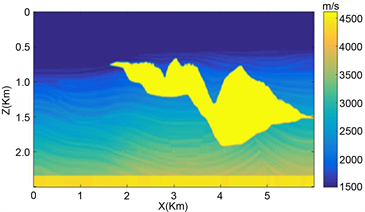(a) Sigsbee真实速度模型(b) Sigsbee初始速度模型

Figure 2. Sigsbee velocity model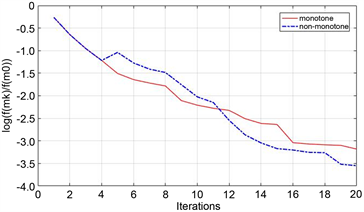(a) 收敛曲线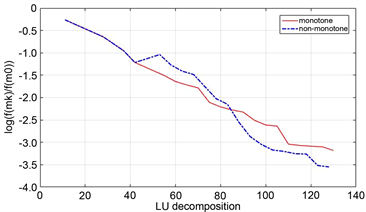(b) 目标泛函对LU分解次数的变化曲线

Figure 3. Curve: The change of the objective function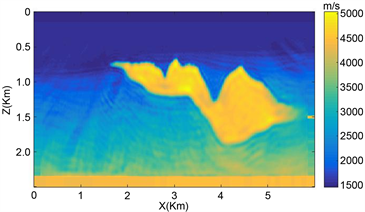(a) CG单调重构结果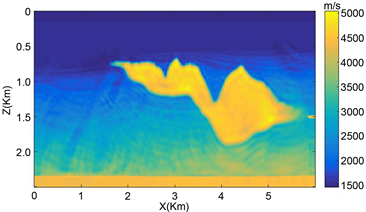(b) CG非单调重构结果

Figure 4. Inversion reconstruction results

Table 1. Comparison between monotone line search method and non-monotone line search method for Sigsbee model in iteration steps, LU decomposition times, $f\left({m}_{k}\right)/f\left({m}_{0}\right)$

4. 结论

FWI反问题是非线性数学物理反问题，其高度非线性使得常规反演方法易陷入局部极值，很难获得高分辨率的反演结果。同时，大规模的计算资源需求给传统反演方法带来了挑战。本文基于线搜索全局化策略、Lanczos对角化方法建立了利用二阶梯度信息的快速CG_NEWTON反演方法。为了进一步提高算法的计算效率，基于伴随方法导出了一种高效计算Hessian阵与向量相乘的方法。基于Sigsbee高模型进行数值实验，数值结果表明基于单调线搜索与非单调线搜索均能有效重构高速度比的盐丘体，说明了CG_NEWTON方法能够充分利用多散射波场，提高重构分辨率；相对于单调线搜索方法，非单调线搜索方法在求解高度非线性FWI反问题时在收敛速度和计算效率方面更加优越。此外，本文给出的方法具有一般性，能适用于其它成像问题。

 Lailly, P. (1983) The Seismic Inverse Problem as a Sequence of Before-Stack Migrations. Conference on Inverse Scat-tering: Theory and Application, Tulsa, 16-18 May 1983, 206-220.

 Tarantola, A. (1984) Inversion of Seismic Re-flection Data in the Acoustic Approximation. Geophysics, 49, 1259-1266.
https://doi.org/10.1190/1.1441754

 Pratt, G.R. (1990) Frequency-Domain Elastic Wave Modeling by Finite Differences: A Tool for Cross-Hole Seismic Imaging. Geophysics, 55, 626-632.
https://doi.org/10.1190/1.1442874

 袁亚湘, 孙文瑜. 最优化理论与方法[M]. 北京: 科学出版社, 1997.

 Brossier, R., Operto, S. and Virieux, J. (2009) Seismic Imaging of Complex Onshore Structures by 2D Elas-tic Frequency-Domain Full-Waveform Inversion. Geophysics, 74, WCC63-WCC76.
https://doi.org/10.1190/1.3215771

 Epanomeritakis, A.V., et al. (2008) A Newton-CG Method for Large-Scale Three-Dimensional Elastic Full-Waveform Seismic Inversion. Inverse Problems, 24, 1-27.
https://doi.org/10.1088/0266-5611/24/3/034015

 Boehm, C. and Ulbrich, M. (2015) A Semi-Smooth New-ton-CG Method for Constrained Parameter Identification in Seismic Tomography. SIAM Journal on Scientific Computing, 37, S334-S364.
https://doi.org/10.1137/140968331

 王义, 董良国. 基于截断牛顿法的VTI介质声波多参数全波形反演[J]. 地球物理学报, 2015, 58(8): 2873-2885.

 Burke, J.V., More, J. and Toranldo, G. (1990) Con-vergence Properties of Trust Region Methods for Linear and Convex Constraints. Mathematical Programming, 47, 305-336.
https://doi.org/10.1007/BF01580867

 Grippo, L., Lampariello, F. and Lucidi, S. (1986) A Non-Monotone Line Search Technique for Newton’s Method. SIAM Journal on Numerical Analysis, 23, 707-716.
https://doi.org/10.1137/0723046

 Toint, P.L. (1996) An Assessment of Non-Monotone Line Search Tech-niques for Unconstrained Optimization. SIAM Journal on Scientific Computing, 17, 725-739.
https://doi.org/10.1137/S106482759427021X

 Dai, Y.H. (2002) On the Non-Monotone Line Search. Optimi-zation Theory and Applications, 112, 315-330.
https://doi.org/10.1023/A:1013653923062

 Zhang, H.C. and Hager, W.W. (2004) A Non-Monotone Line Search Technique and Its Application to Unconstrained Optimization. SIAM Journal on Optimization, 14, 1043-1056.
https://doi.org/10.1137/S1052623403428208

 Metivier, L., Brossier, R., Virieux, J., et al. (2013) Full Wave-form Inversion and the Truncated Newton Method. SIAM Journal on Scientific Computing, 35, B401-B437.
https://doi.org/10.1137/120877854

 Metivier, L., Bretaudeau, F., Brossier, R., et al. (2014) Full Waveform In-version and the Truncated Newton Method: Quantitative Imaging of Complex Subsurface Structures. Geophysical Pro-specting, 62, 1353-1375.
https://doi.org/10.1111/1365-2478.12136

 Yang, P., Brossier, R., Metivier, L., et al. (2018) A Time-Domain Preconditioned Truncated Newton Approach to Visco-Acoustic Multi-Parameter Full Waveform Inversion. SIAM Jour-nal on Scientific Computing, 40, B1101-B1130.
https://doi.org/10.1137/17M1126126

 Paffenholz, J., Mclain, B., Zaske, J., et al. (2002) Subsalt Multiple At-tenuation and Imaging: Observations from the Sigsbee2B Synthetic Dataset. 82nd Annual International Meeting, SEG, Expanded Abstracts, Salt Lake City, 6-11 October 2002, 2122-2125.
https://doi.org/10.1190/1.1817123

 Paige, C.C. and Saunders, M.A. (1975) Solution of Sparse Indefinite Systems of Linear Equations. SIAM Journal on Numerical Analysis, 12, 617-629.
https://doi.org/10.1137/0712047

 He, Q.H. and Wang, Y.F. (2020) Inexact New-ton-Type Methods Based on Lanczos Orthonormal Method and Application for Full Waveform Inversion. Inverse Prob-lems, 36, Article ID: 115007.
https://doi.org/10.1088/1361-6420/abb8ea

 Berenger, J.P. (1994) A Perfectly Matched Layer for the Absorp-tion of Electromagnetic Waves. Journal of Computational Physics, 114, 185-200.
https://doi.org/10.1006/jcph.1994.1159

 Hustedt, B., Operto, S. and Virieux, J. (2014) Mixed-Grid and Stag-gered-Grid Finite-Difference Methods for Frequency-Domain Acoustic Wave Modelling. GeophysicalJournal Interna-tional, 157, 1269-1296.
https://doi.org/10.1111/j.1365-246X.2004.02289.x

 Ghysels, P., Li, X.S., Rouet, F.-H., et al. (2016) An Effi-cient Multicore Implementation of a Novel HSS-Structured Multi-Frontal Solver Using Randomized Sampling. SIAM Journal on Scientific Computing, 38, S358-S384.
https://doi.org/10.1137/15M1010117

Top